# Lower Duncan River Kokanee AUC Analysis 2013

The suggested citation for this analytic report is:

Thorley, J.L. and Hogan, P.M. (2015) Lower Duncan River Kokanee AUC Analysis 2013. A Poisson Consulting Analysis Report. URL: https://www.poissonconsulting.ca/f/577555490.

## Background

Since 2008 aerial surveys have been conducted in the Lower Duncan River (LDR) to count the number of kokanee spawners. The primary objectives of the current analysis report are to

• Estimate the aerial observer efficiency based on ground counts
• Estimate the annual kokanee spawner abundance from the aerial counts
• Estimate the peak spawn timing

## Methods

### Data

The data were provided by LGL Limited in the form of an Access database and an Excel spreadsheet.

### Analysis

Hierarchical Bayesian models were fitted to the LDR kokanee enumeration data using R version 3.0.2 (Team, 2013) and JAGS 3.3.0 (Plummer, 2012) which interfaced with each other via jaggernaut 1.6 (Thorley, 2014). For additional information on hierarchical Bayesian modelling in the BUGS language, of which JAGS uses a dialect, the reader is referred to Kery and Schaub (2011) pages 41-44.

Unless specified, the models assumed vague (low information) prior distributions (Kéry and Schaub, 2011, p. 36). The posterior distributions were estimated from a minimum of 1,000 Markov Chain Monte Carlo (MCMC) samples thinned from the second halves of three chains (Kéry and Schaub, 2011, pp. 38-40). Model convergence was confirmed by ensuring that Rhat (Kéry and Schaub, 2011, p. 40) was less than 1.1 for each of the parameters in the model (Kéry and Schaub, 2011, p. 61). Model adequacy was confirmed by examination of residual plots.

The posterior distributions of the fixed (Kéry and Schaub, 2011, p. 75) parameters are summarised in terms of a point estimate (mean), lower and upper 95% credible limits (2.5th and 97.5th percentiles), the standard deviation (SD), percent relative error (half the 95% credible interval as a percent of the point estimate) and significance (Kéry and Schaub, 2011, p. 37,42).

The results are displayed graphically by plotting the modeled relationships between particular variables and the response with 95% credible intervals (CRIs) with the remaining variables held constant. In general, continuous and discrete fixed variables are held constant at their mean and first level values respectively while random variables are held constant at their typical values (expected values of the underlying hyperdistributions) (Kéry and Schaub, 2011, pp. 77-82). Where informative the influence of particular variables is expressed in terms of the effect size (i.e., percent change in the response variable) with 95% credible intervals (Bradford et al. 2005). Plots were produced using the ggplot2 R package (Wickham, 2009).

### Observer Efficiency

The aerial observer efficiency was estimated from ground counts using a Poisson model. Key assumptions of the observer efficiency model include:

• The spawner abundance at a site does not change between the ground and aerial count.
• The ground observers are unbiased with the ground count being drawn from a Poisson distribution.
• The aerial observer efficiency does not vary systematically with abundance or channel type, i.e., sidechannel versus main channel.
• The aerial counts are drawn from an overdispersed Poisson distribution.

### Area-Under-the-Curve

The aerial spawner counts were analysed using a hierarchical Bayesian Area-Under-the-Curve (AUC) model (Hilborn et al. 1999). Key assumptions of the AUC model include:

• Spawner arrival and departure are normally distributed (Hilborn et al. 1999)
• The duration of spawning is constant across years
• The peak spawn timing in each year is drawn from a normal distribution (Su et al. 2001)
• The residence time is between 7 and 14 days for spawners (Acara, 1970; Morbey and Ydenberg, 2003)
• The observer efficiency is described by a normal distribution with a mean of 1.07 and SD of 0.19 and lower and upper limits of 0.75 and 1.5 as estimated by the observer efficiency model
• The residual variation in the spawner counts is normally distributed

### Model Code

The first three tables describe the JAGS distributions, functions and operators used in the models. For additional information on the JAGS dialect of the BUGS language see the JAGS User Manual (Plummer, 2012).

#### JAGS Distributions

Distribution Description
dgamma(shape, rate) Gamma distribution
dnorm(mu, sd^-2) Normal distribution
dpois(lambda) Poisson distribution
dunif(a, b) Uniform distribution

#### JAGS Functions

Function Description
length(x) Length of vector x
log(x) Natural logarithm of x
phi(x) Standard normal cumulative distribution function for x
T(x,y) Truncate distribution so that values lie between x and y

#### JAGS Operators

Operator Description
<- Deterministic relationship
~ Stochastic relationship
1:n Vector of integers from 1 to n
a[1:n] Subset of first n values in a
for (i in 1:n) {...} Repeat for 1 to n times incrementing i each time
x^y Power where x is raised to the power of y

### Observer Efficiency

Variable/Parameter Description
Aerial[i] Aerial count for ith survey
bAbundance Intercept for log(eAbundance)
bDispersion Variation in eDispersion
bEfficiency Intercept for eEfficiency
eAbundance[i] Predicted spawner abundance for ith survey
eAerial[i] Predicted aerial count for ith survey
eEfficiency[i] Predicted aerial observer efficiency for ith survey
Ground[i] Ground count for ith survey
sAbundance SD of log(eAbundance)

#### Observer Efficiency - Model 1

model {

bEfficiency ~ dunif(0, 3)

bAbundance ~ dnorm(5, 5^-2)
sAbundance ~ dunif(0, 5)

bDispersion ~ dgamma(0.1, 0.1)
for (i in 1:length(Aerial)) {
eEfficiency[i] <- bEfficiency

eAbundance[i] ~ dlnorm(bAbundance, sAbundance^-2)
Ground[i] ~ dpois(eAbundance[i])

eAerial[i] <- eAbundance[i] * eEfficiency[i]
eDispersion[i] ~ dgamma(bDispersion, bDispersion)
Aerial[i] ~ dpois(eAerial[i] * eDispersion[i])
}
}

### Area-Under-The-Curve

Variable/Parameter Description
bAbundance Intercept for log(eAbundance)
bAbundanceYear[i] Effect of ith year on log(eAbundance)
bDuration Intercept for eDuration
bEfficiency Intercept for eEfficiency
bPeakTiming Intercept for ePeakTiming
bPeakTimingYear[i] Effect of ith year on ePeakTiming
bResidenceTime Spawner residence time
Count[i] Spawner count on ith survey
Dayte[i] Centred day of the year of ith survey
eAbundance[i] Predicted annual spawner abundance for ith survey
eCount[i] Predicted spawner count on ith survey
eDuration[i] Expected SD of the duration of spawner arrival timing
eEfficiency[i] Predicted aerial observer efficiency ith survey
ePeakTiming[i] Predicted timing of annual peak spawner abundance for ith survey
eSpawners[i] Predicted number of spawners on ith survey
sCount SD of log(eSpawners)

#### Area-Under-The-Curve - Model 1

model {

bEfficiency ~ dnorm(1.07, 0.19^-2) T(0.75, 1.5)

bAbundance ~ dnorm(10, 5^-2)
bPeakTiming ~ dnorm(0, 5)
bDuration ~ dunif(0, 42)

sAbundanceYear ~ dunif(0, 5)
sPeakTimingYear ~ dunif(0, 28)
for (i in 1:nYear) {
bAbundanceYear[i] ~ dnorm (0, sAbundanceYear^-2)
bPeakTimingYear[i] ~ dnorm (0, sPeakTimingYear^-2)
}

bResidenceTime ~ dunif(7, 14)

sCount ~ dunif(0, 10000)
for (i in 1:length(Count)) {

log(eAbundance[i]) <- bAbundance
+ bAbundanceYear[Year[i]]

ePeakTiming[i] <- bPeakTiming
+ bPeakTimingYear[Year[i]]

eDuration[i] <- bDuration

eSpawners[i] <- (phi((Dayte[i] - (ePeakTiming[i] - bResidenceTime/2))
/ eDuration[i])
- phi((Dayte[i] - (ePeakTiming[i] + bResidenceTime/2))
/ eDuration[i]))
* eAbundance[i]

eEfficiency[i] <- bEfficiency

eCount[i] <- eSpawners[i] * eEfficiency[i]
Count[i] ~ dnorm(eCount[i], sCount^-2)
}
} 

## Parameter Estimates

The posterior distributions for the fixed (Kery and Schaub 2011 p. 75) parameters in each model are summarised below.

### Observer Efficiency

Parameter Estimate Lower Upper SD Error Significance
bAbundance 5.094 3.914 6.301 0.6076 23 0
bDispersion 3.617 1.129 7.692 1.7146 91 0
bEfficiency 1.071 0.751 1.500 0.1901 35 0
sAbundance 1.890 1.153 3.089 0.5120 51 0
Rhat Iterations
1.1 40000

### Area-Under-The-Curve

Parameter Estimate Lower Upper SD Error Significance
bAbundance 1.025e+01 8.5323 11.468 0.6888 14 0.0000
bDuration 6.155e+00 3.0483 8.540 1.3507 45 0.0000
bEfficiency 1.071e+00 0.7880 1.395 0.1632 28 0.0000
bPeakTiming 9.212e-02 -0.7447 1.006 0.4385 950 0.8703
bResidenceTime 1.068e+01 7.2022 13.847 2.0605 31 0.0000
sAbundanceYear 1.166e+00 0.4239 3.204 0.6994 119 0.0000
sCount 6.495e+03 4819.4913 8677.541 992.4000 30 0.0000
sPeakTimingYear 1.013e+01 4.3513 22.884 4.7219 92 0.0000
Rhat Iterations
1.03 1e+05

## Figures

### Observer Efficiency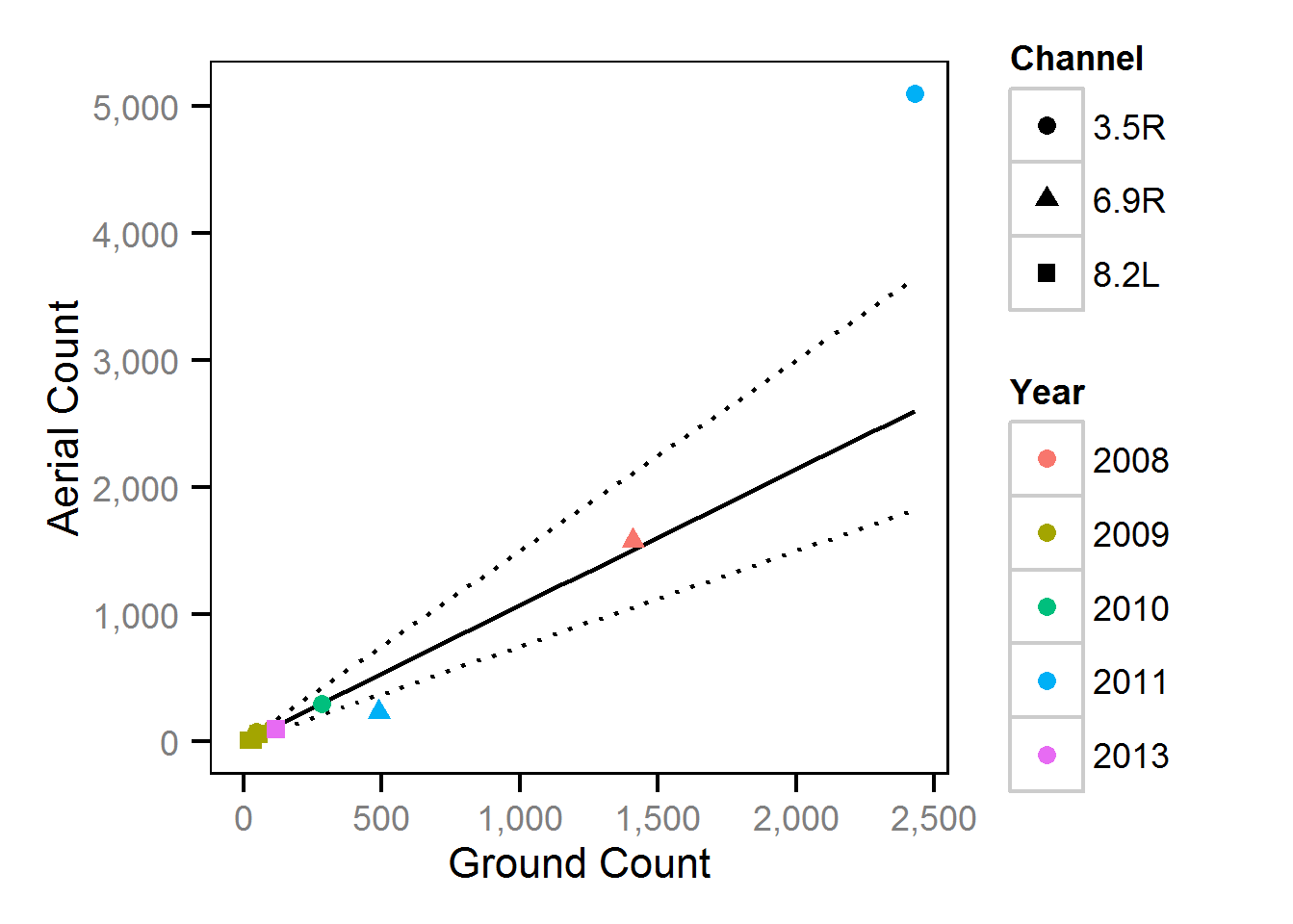Figure 1. Aerial versus ground kokanee spawner counts and predicted ground count (with 95% CRIs) by year and channel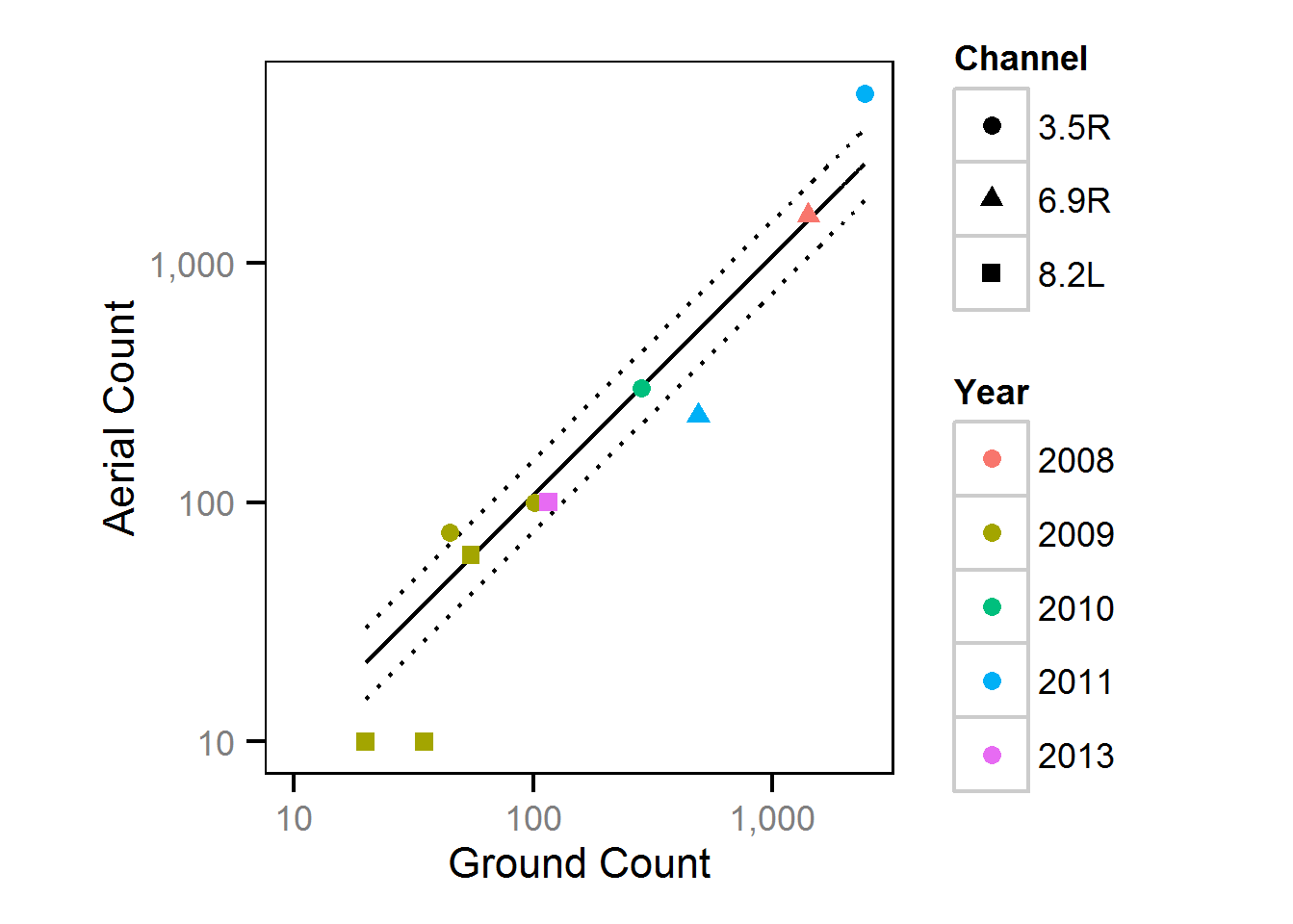Figure 2. Aerial versus ground kokanee spawner counts (on log10 scales) and predicted ground count (with 95% CRIs) by year and channel

### Area-Under-The-Curve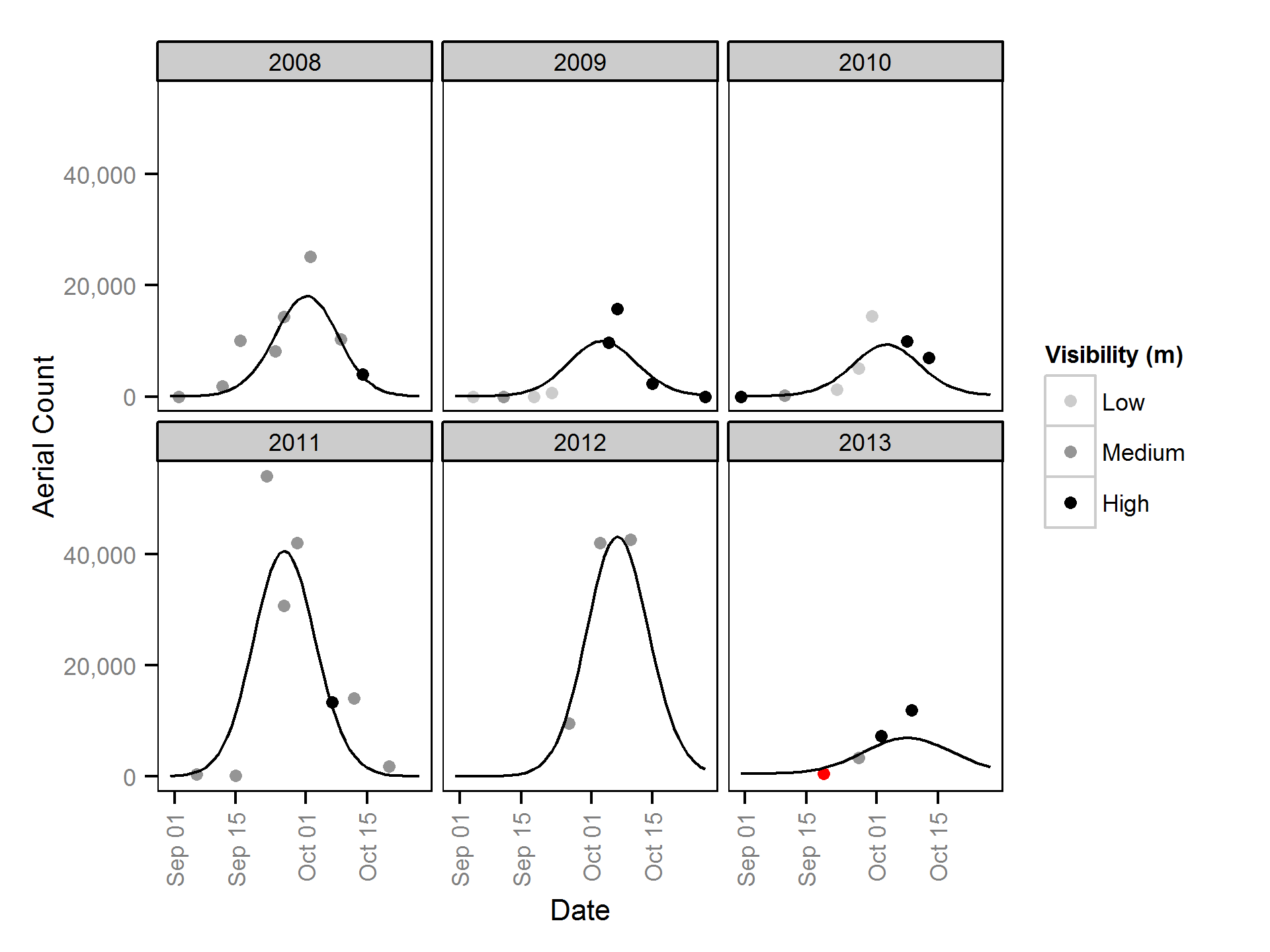Figure 3. Kokanee spawner aerial counts with predicted aerial counts by date and year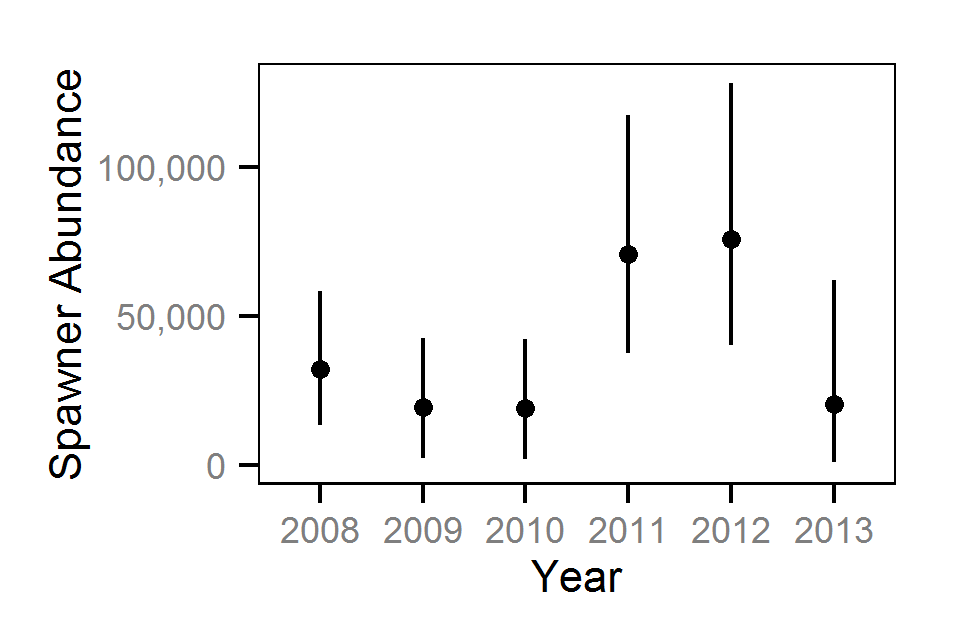Figure 4. Predicted total kokanee spawner abundance by year with 95% CRIs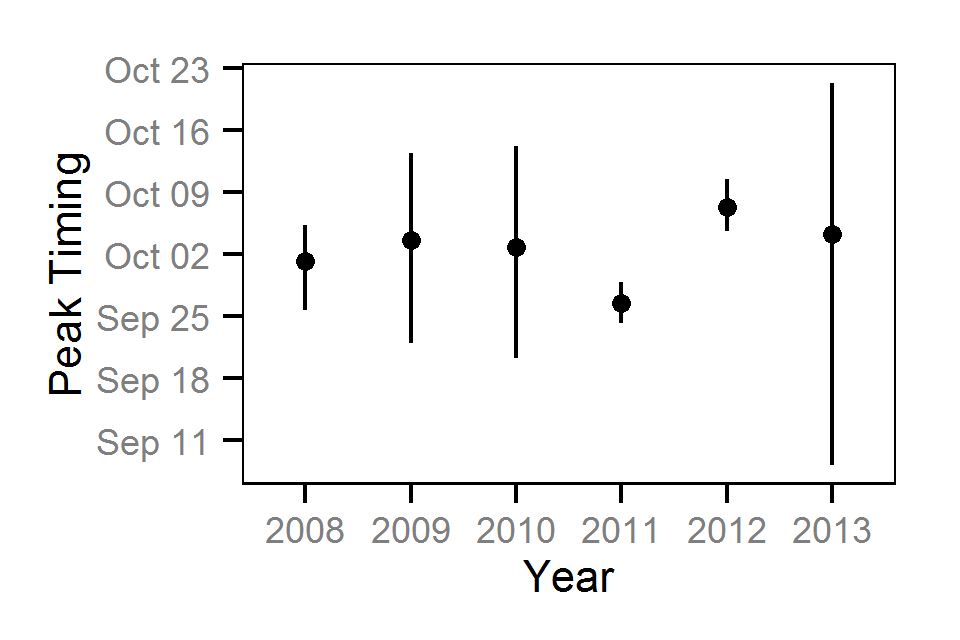Figure 5. Predicted kokanee peak spawn timing by year with 95% CRIs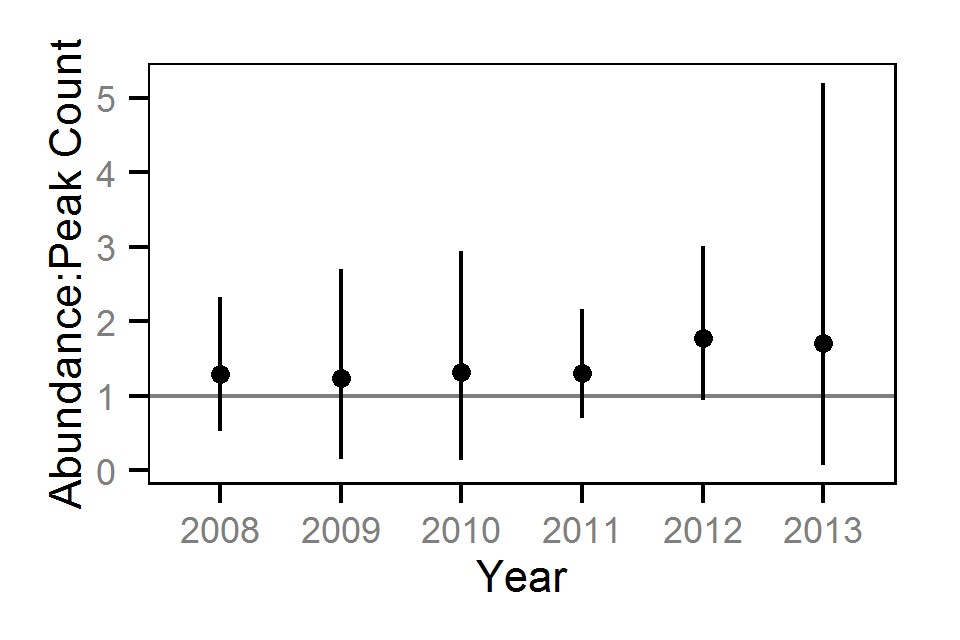Figure 6. Predicted total kokanee spawner abundance to peak count ratio by year with 95% CRIs

## Acknowledgements

This analysis report was made possible through the contributions of the following organisations and individuals:

• BC Hydro
• Guy Martel
• Okanagan National Alliance
• Michael Zimmer
• Natasha Audy
• Skyeler Folks
• LGL Limited
• Elmar Plate
• Amec Earth and Environmental
• Louise Porto
• Crystal Lawrence
• Clint Tarala
• Poisson Consulting Ltd.
• Robyn Irvine